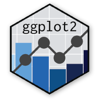Show Sidebar Hide Sidebar# geom_histogram in ggplot2

How to make a histogram in ggplot2. Examples and tutorials for plotting histograms with geom_histogram, geom_density and stat_density.

### New to Plotly?

Plotly's R library is free and open source!
You can set up Plotly to work in online or offline mode.
We also have a quick-reference cheatsheet (new!) to help you get started!

### Version Check

Version 4 of Plotly's R package is now available!
Check out this post for more information on breaking changes and new features available in this version.

library(plotly)
packageVersion('plotly')

##  '4.9.1'


### Basic Histogram

library(plotly)

dat <- data.frame(xx = c(runif(100,20,50),runif(100,40,80),runif(100,0,30)),yy = rep(letters[1:3],each = 100))

p <- ggplot(dat,aes(x=xx)) +
geom_histogram(data=subset(dat,yy == 'a'),fill = "red", alpha = 0.2) +
geom_histogram(data=subset(dat,yy == 'b'),fill = "blue", alpha = 0.2) +
geom_histogram(data=subset(dat,yy == 'c'),fill = "green", alpha = 0.2)

p <- ggplotly(p)

p


library(plotly)

df1 <- data.frame(cond = factor( rep(c("A","B"), each=200) ),
rating = c(rnorm(200),rnorm(200, mean=.8)))

df2 <- data.frame(x=c(.5,1),cond=factor(c("A","B")))

p <- ggplot(data=df1, aes(x=rating, fill=cond)) +
geom_vline(xintercept=c(.5,1)) +
geom_histogram(binwidth=.5, position="dodge")

p <- ggplotly(p)

p


library(plotly)

df <- data.frame (type=rep(1:2, each=1000), subtype=rep(c("a","b"), each=500), value=rnorm(4000, 0,1))

library(plyr)
df.text<-ddply(df,.(type,subtype),summarise,mean.value=mean(value))

p <- ggplot(df, aes(x=value, fill=subtype)) +
geom_histogram(position="identity", alpha=0.4)+
facet_grid(. ~ type)

p <- ggplotly(p)

p


### Probability & Density

library(plotly)

df <- data.frame(x = rnorm(1000))

p <- ggplot(df, aes(x=x)) +
geom_histogram(aes(y = ..density..), binwidth=density(df$x)$bw) +
geom_density(fill="red", alpha = 0.2)

p <- ggplotly(p)

p